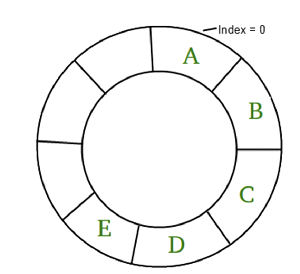# Maximum sum in circular array such that no two elements are adjacent in C++

In this problem, we are given a circular array cirArr[]. Our task is to create a program to find the Maximum sum in circular array such that no two elements are adjacent in C++.

## Problem Description

For the circular array, we need to find the maximum sum sum of elements of the array such that adjacent elements cannot be taken i.e. we need to take alternate elements.

Circular Array is a special type of array in which the last element of the array is connected to the first element.Let’s take an example to understand the problem,

## Input

cirArr[] = {4, 1, 5, 3, 2}

## Output

9

## Explanation

The maximum sum circular subsequence is [4, 5, 2]. Sum = 9

## Solution Approach

The solution to the problem is using a dynamic programming approach to find the maximum sum. The sum can be extracted by treating the circular array as two arrays, one from index 0 to N-2 and other from index 1 to n-1. This will create two arrays and the maximum sum out the sums from these array’s will be the result.

## Example

Program to illustrate the working of our solution,

Live Demo

#include <iostream>
using namespace std;
int calcMaxVal(int a, int b){
if(a > b)
return a;
return b;
}
int calcMaxSumSubSeq(int cirArr[], int start, int end, int n) {
int DP[n];
int maxSum = 0;
for (int i = start; i < (end + 1); i++) {
DP[i] = cirArr[i];
if (maxSum < cirArr[i])
maxSum = cirArr[i];
}
for (int i = (start + 2); i < (end + 1); i++) {
for (int j = 0; j < i - 1; j++) {
if (DP[i] < DP[j] + cirArr[i]) {
DP[i] = DP[j] + cirArr[i];
if (maxSum < DP[i])
maxSum = DP[i];
}
}
}
return maxSum;
}
int findMaxSum(int cirArr[], int n){
int maxSumArray1 = calcMaxSumSubSeq(cirArr, 0, (n-2), n);
int maxSumArray2 = calcMaxSumSubSeq(cirArr, 1, (n-1), n);
int maxSum = calcMaxVal(maxSumArray1, maxSumArray2);
return maxSum;
}
int main(){
int cirArr[] = {4, 1, 5, 3, 2};
int n = sizeof(cirArr)/sizeof(cirArr);
cout<<"The maximum sum in circular array such that no two elements are adjacent is "<<findMaxSum(cirArr, n);
return 0;
}

## Output

The maximum sum in circular array such that no two elements are adjacent is 9